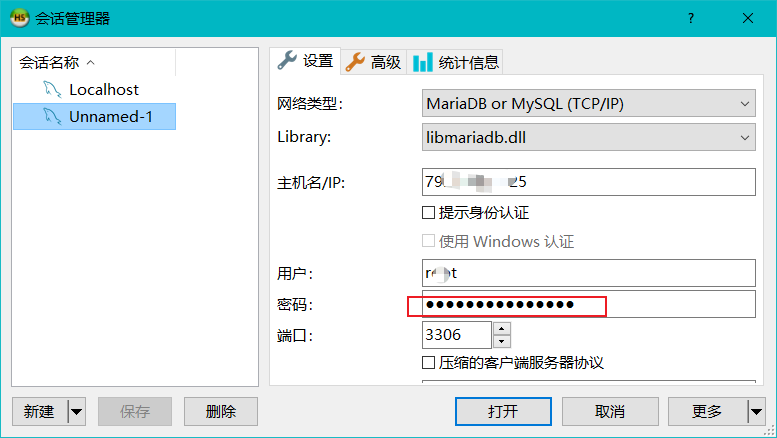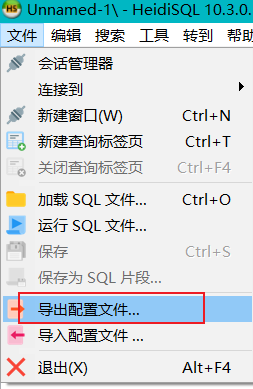# 获取heidisql保存的密码

1.heidsql 默认保存的密码忘记了，如何找回？2.连接进入数据库，导出配置文件。3. 使用下列python代码 运行

```import re
settings = r"D:\123.txt" #配置文件保存的位置

with open(settings,encoding="utf8") as f:
passwords = [re.split("\<\|\|\|\>",r)[-1] for r in lines]

def heidipass(code):
ascii = code[:-1]
d = int(code [-1])
decode = lambda x:chr(int(x,16) - d)

print(heidipass(r))```